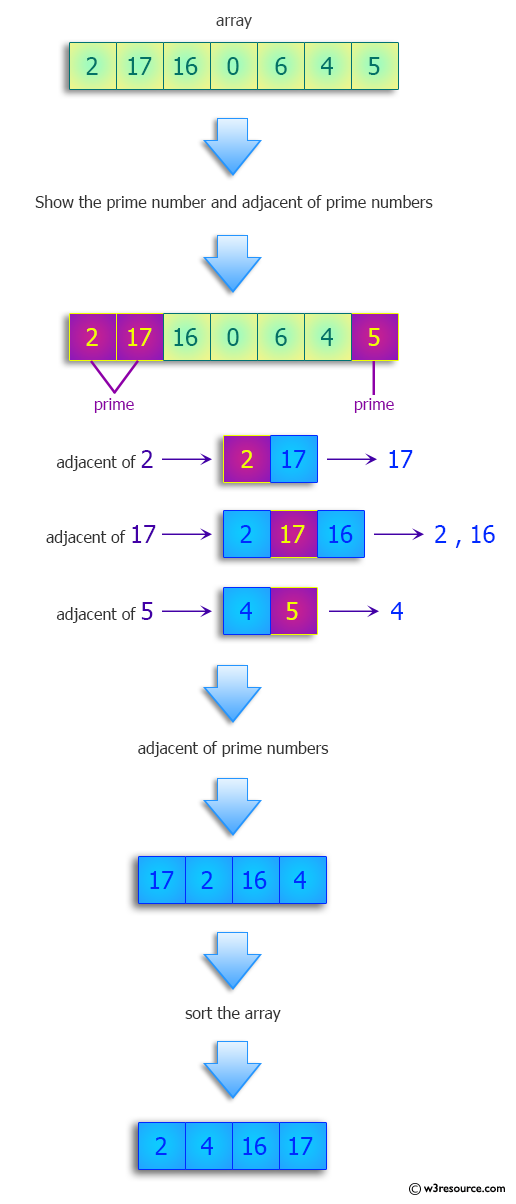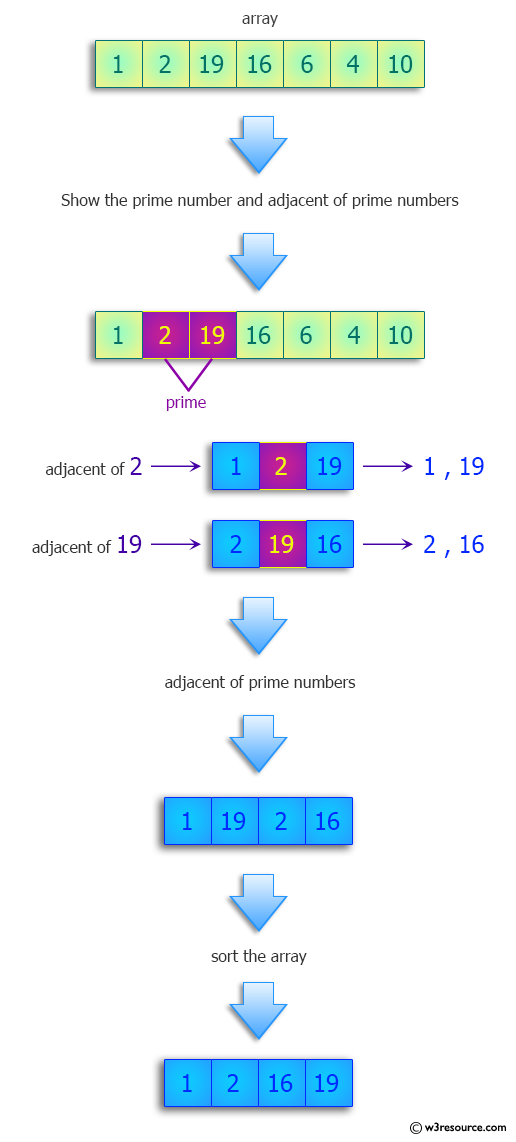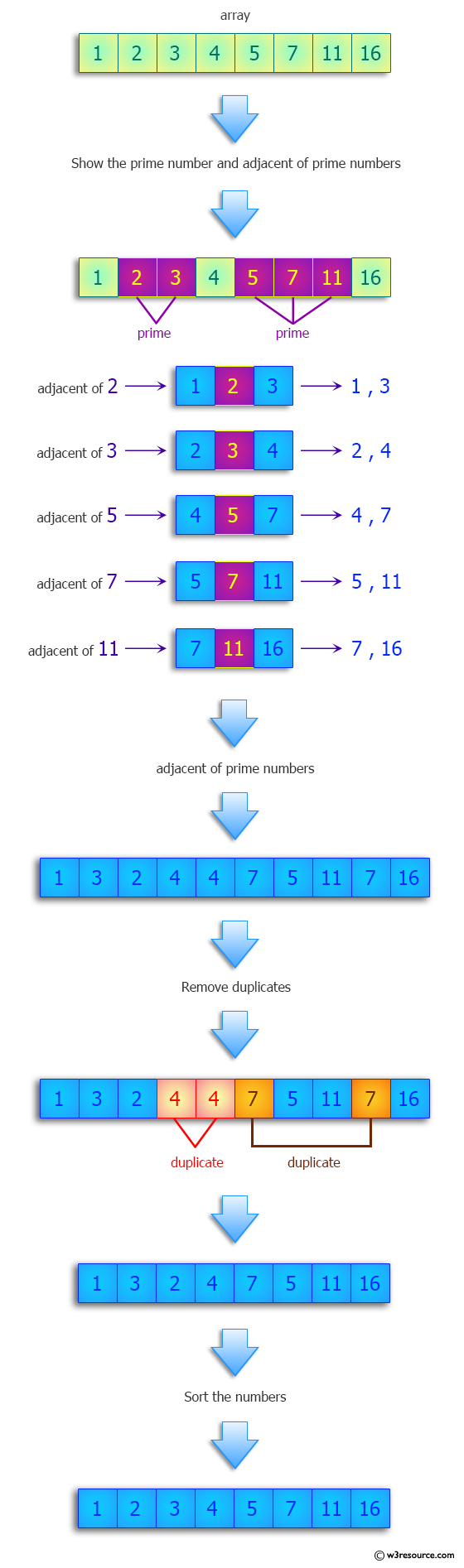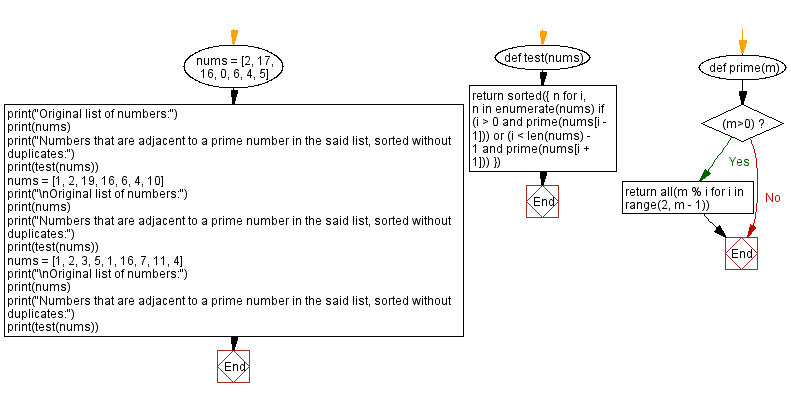﻿ Python: Find numbers that are adjacent to a prime number in the list, sorted without duplicates - w3resource# Python: Find numbers that are adjacent to a prime number in the list, sorted without duplicates

## Python Programming Puzzles: Exercise-60 with Solution

Prime number: A number that is divisible only by itself and 1 (e.g. 2, 3, 5, 7, 11).
Write a Python program to find a list of all numbers that are adjacent to a prime number in the list, sorted without duplicates.

```Input:
[2, 17, 16, 0, 6, 4, 5]
Output:
[2, 4, 6, 16, 17]

Input:
[1, 2, 19, 16, 6, 4, 10]
Output:
[1, 2, 16, 19]

Input:
[1, 2, 3, 5, 1, 16, 7, 11, 4]
Output:
[1, 2, 3, 4, 5, 7, 11, 16]
```

Pictorial Presentation:Sample Solution:

Python Code:

``````#License: https://bit.ly/3oLErEI

def test(nums):
return sorted({
n for i, n in enumerate(nums)
if (i > 0 and prime(nums[i - 1])) or (i < len(nums) - 1 and prime(nums[i + 1]))
})

def prime(m):
if (m>0):
return all(m % i for i in range(2, m - 1))

nums = [2, 17, 16, 0, 6, 4, 5]
print("Original list of numbers:")
print(nums)
print("Numbers that are adjacent to a prime number in the said list, sorted without duplicates:")
print(test(nums))
nums = [1, 2, 19, 16, 6, 4, 10]
print("\nOriginal list of numbers:")
print(nums)
print("Numbers that are adjacent to a prime number in the said list, sorted without duplicates:")
print(test(nums))
nums = [1, 2, 3, 5, 1, 16, 7, 11, 4]
print("\nOriginal list of numbers:")
print(nums)
print("Numbers that are adjacent to a prime number in the said list, sorted without duplicates:")
print(test(nums))
``````

Sample Output:

```Original list of numbers:
[2, 17, 16, 0, 6, 4, 5]
Numbers that are adjacent to a prime number in the said list, sorted without duplicates:
[2, 4, 16, 17]

Original list of numbers:
[1, 2, 19, 16, 6, 4, 10]
Numbers that are adjacent to a prime number in the said list, sorted without duplicates:
[1, 2, 16, 19]

Original list of numbers:
[1, 2, 3, 5, 1, 16, 7, 11, 4]
Numbers that are adjacent to a prime number in the said list, sorted without duplicates:
[1, 2, 3, 4, 5, 7, 11, 16]
```

Flowchart:## Visualize Python code execution:

The following tool visualize what the computer is doing step-by-step as it executes the said program:

Python Code Editor :

Have another way to solve this solution? Contribute your code (and comments) through Disqus.

What is the difficulty level of this exercise?

Test your Programming skills with w3resource's quiz.

﻿

## Python: Tips of the Day

Clamps num within the inclusive range specified by the boundary values x and y:

Example:

```def tips_clamp_num(num,x,y):
return max(min(num, max(x, y)), min(x, y))
print(tips_clamp_num(2, 4, 6))
print(tips_clamp_num(1, -1, -6))
```

Output:

```4
-1
```